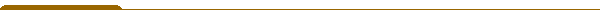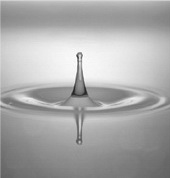Course materials Jump to:Contents: First-order equations (the methof of characteristics, Cauchy problem for quasi-linear and fully non-linear equations, weak solutions). General techniques and principles for second-order equations The wave equation (d'Alembert's formula, weak solutions, reflections, Duhamel's principle, two-dimensional and three-dimensional wave equation, conservation of energy). The Laplace equation (Mean Value Theorem and Maximum Principle, the fundamental solution, Green's function, Poisson kernel). The heat equation (Weak maximum principle, properties of the heat kernel, properties of the solution to the pure initial value problem). Textbooks: Robert C. McOwen, Partial Differential Equations, Methods and Applications, Prentice Hall/Pearson Education, Inc., 2003 (Second Edition) * Lawrence C. Evans. Partial Differential Equations, AMS, Providence, RI. Series: Graduate Studies in Mathematics, Vol. 19, 1998. Instruction: Lectures and problem solving sessions. Examination: Written examination at the end of the course.